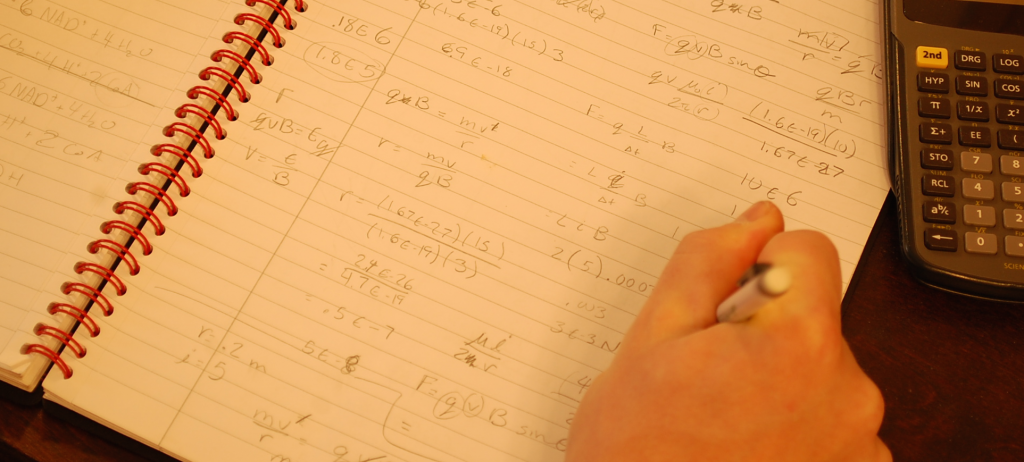Charlottesville
• (434) 296-5111
Great Falls
• (703) 759-3624
McLean
• (703) 288-2808# SAT Math

The SAT Math Test will assess students’ understanding of high school math concepts, with a strong emphasis on algebra. We can classify the questions students will see into four content areas:

• Problem Solving and Data Analysis (e.g., percents, proportions, basic statistics)
• Heart of Algebra (ex. algebraic equations, systems of equations, graphs of linear equations, linear models)
• Additional Topics (e.g., angles, triangles, circles, trigonometry, complex numbers)

• The two math sections – No Calculator and Calculator – will appear back-to-back at the end of the test. The No Calculator section will require students to do some manual calculations, so students should practice doing as many math questions without the help of a calculator as they can. In general, questions in both math sections progress in order of increasing difficulty, although all questions are worth the same.

The No Calculator section will have 5 free response questions and the Calculator section will have 8 free response questions. In preparing for the SAT, students should understand the rules for gridding in their numerical answers. It’s also a useful strategy to try answering multiple choice questions without looking at the answer choices as preparation for the free response questions.

## GLC’s Tips for the SAT Math Test

DO know how to answer math questions without the help of a calculator.
You should know how to do long division, double digit multiplication, addition and subtraction of fractions, and conversion between fractions and decimals without the help of a calculator.

DO use GLC’s test taking strategies.
Try plugging answer choices into the question or picking numbers for questions with 2 variables.

Since you’re not penalized for wrong answers, select an answer for each question, even ones you don’t know.

The directions for each test section are the same on each SAT. Read the directions on your first practice test, remember them, and then don’t waste time reading them on the actual test.

DON’T get bogged down on any one question.
If you’ve spent over a minute on a question and you’re not close to the answer, move to the next question.

DON’T overuse your calculator on the Math with Calculator section.
You might be excited to use you calculator again, but many questions on the Calculator section can be solved faster in your head or on paper than on the calculator, so don’t feel like you must use it on every question.

## GLC’s Approach to SAT Math

The SAT Math Test can be challenging because it combines a wide variety of concepts with problem-solving skills in a condensed timeframe. In our prep, we prioritize fundamental concepts in a logical progression and teach a problem-solving approach that can boost results on this difficult test.

### Building Strong Foundations

The SAT doesn’t test math content that’s especially complicated or foreign, but more than ever, students will have to remember how to do multi-step math problems exactly the same way that they learned in class. The kicker is that it may have been many months or even years since students learned how to do these problems. It can be especially difficult for juniors and seniors in more advanced math classes to recall older concepts like how to put a quadratic function in vertex form, so our SAT curriculum provides a thorough review on the skills that slow students down the most when they’re rusty. Our preparation also devotes time to working out some of the most detailed and complex problems in the Passport to Advanced Math sub-section since students benefit most from doing these problems with an instructor.

### Strategic Time Management

Complex math problems on the SAT are dangerous because they’re usually so solvable. Students might get halfway through a problem before getting stuck and realizing that it will take five minutes to solve—time they can’t afford when the clock is ticking. We teach students how to identify complicated problems and work around them so they maximize the number of “easy points” they earn on each section, an approach that gets strong results while reducing stress.

### Developing Problem Solvers

Once students know the math concepts tested on the SAT, attention should turn to problem solving skills because the SAT loves to ask simple questions in confusing ways. We use real SAT example problems to demonstrate how to dissect verbose word problems, break diagrams into manageable chunks, and use questions against themselves in the search for solutions.

SAT tutoring helps students learn the fundamental concepts, problem solving skills, and confidence needed for top math scores. Click the button below to learn more about our programs, view class schedules, or register for SAT Prep.